# SAT Math Multiple Choice Question 825: Answer and Explanation

### Test Information

Question: 825

15.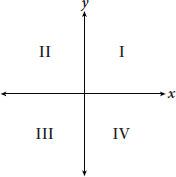If the system of inequalities given by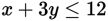and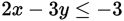is graphed on the coordinate plane above, which quadrant of the plane contains nosolutions to the system?

• C. Quadrants I and IV
• D. Quadrants III and IV

Explanation:

B

Difficulty: Hard

Category: Heart of Algebra / Inequalities

Strategic Advice: The only way to solve a system of inequalities is to graph it, so draw a sketch on the coordinate plane provided to find the answer. Remember, you are looking for the quadrant that contains no solutions to the system.

Getting to the Answer: Start by rewriting each inequality in slope-intercept form, y = mx + b, where m is the slope and b is the y-intercept. Then, use m and b to graph each inequality.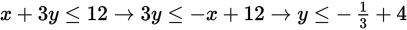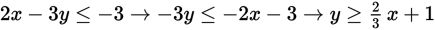To represent the first inequality, shade below its line (≤); to represent the second inequality, shade above its line (≥). The solution to the system of inequalities is the region where the two shaded areas overlap. A sample graph follows: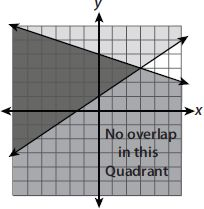Notice that there is some darker shading (the overlap) in quadrants I, II, and III, making quadrant IV the only one that does not contain solutions to the system. Choice (B) is correct.